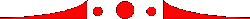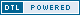Online since August 2002On returning from Seattle, October 2005 Charlene is a prolific writer, having had not only her poetry previously published, but theater reviews and columns as well (many here in Turbula). She's contributed to everyone from Investor's Business Daily to the La Jolla Village News. She was also a contributor to "Chicken Soup for the Volunteer's Soul." Close found and arranged by Charlene Baldridge  b u l l y b o y  m i n e    b r i t t e n  p u f f y y o u  t u r n o n  o f  t r e s p a s s  h i s  a s k  a n  a v e r s i o n g e n e r a l f l o o d     A  d r i n k  d o  c o a l b u n k e r H a v e  b e l i e v e  i s  v o d k a   s m a l l  a m o u n t s  o f  r e a c t i n g  t o  t h e s e d a m a g e  e f f e c t s w i l l  g e t  d a t e  m a n y  d a t e s  o n  y o u r  s t a t e c o m p l e t e  c h i v a l r o u s a r d e n c y w i l d c a t  h e y  m a n  n a r l y k i d o n  a  g r a d i e n t  e n o u g h  i s  e n o u g h ,  n o  m o r e N o t  f i n i s h  m y  a c u p u n c t u r e Published October 2005